The purpose of this lesson is to compare the simulation results of the VCSEL laser component with the published articles  and .

The first part of the lesson will compare the LI and IV curves of the component for different input parameters with the experiments presented in .

The second part of the lesson will demonstrate the dynamic behavior of the component using the parameters from .

## Creating a Project

Using the default global parameters, we can start adding the components to design the basic VCSEL transmitter.

From the component library drag and drop the following component sin to the layout:

• From “Default/Transmitters Library/Bit Sequence Generators”, drag and drop the “Pseudo-Random Bit Sequence Generator” into the layout.
• From “Default/Transmitters Library/Pulse Generators/Electrical”, drag and drop the “NRZ Pulse Generator” into the layout.
• From “Default/Transmitters Library/Optical Sources”, drag and drop the “VCSEL Laser” into the layout.
• From “Default/Visualizers Library/Electrical”, drag and drop the “Oscilloscope Visualizer” into the layout.
• From “Default/Visualizers Library/Optical”, drag and drop the “Optical Time Domain Visualizer” into the layout.
• From “Default/Visualizers Library/Optical”, drag and drop the “Optical Spectrum Analyzer” into the layout.

The next step is to connect the components according to the Figure 1.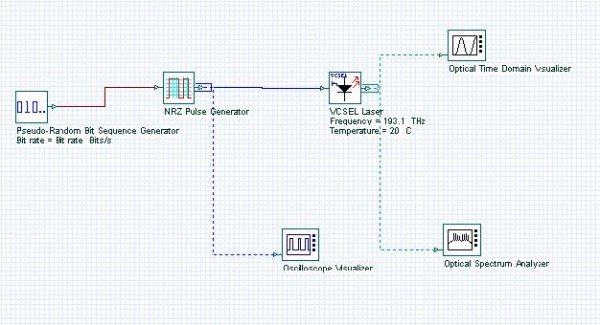Figure 1: VCSEL transmitter

## First device: 863 nm laser

Parameter setup

The laser parameters of OptiSystem VCSEL component should be the same as the 863 nm VCSEL from .

 Parameter Value Frequency 863 nm Thermal impedance 2600 Thermal time constant 1e-006 Scaling factor 2.6e-008 Gain coefficient 16000 Carrier number at transparency 19400000 Carrier lifetime 5e-009 Photon lifetime 2.28e-012 Spontaneous emission factor 1e-006 Injection efficiency 1 Max input current 40 a – Ioff(T) 1.246e-3, -2.545e-5, 2.908e-7, -2.531e-10, 1.022e-12 b – V(T) 1 c – V(I) 1.721 275, -2.439e4, 1.338e6, -4.154e7, 6.683e8, -4.296e9

Table 1: 863 nm VCSEL parameters

For this example, the parameter fitting parameter is disabled because we want to use the same parameters from .

If the parameter fitting is enabled, the component will recalculate new parameters and they may differ from .

We also enabled the graph calculation in order to compare the calculated LI and IV curves with the measurements.

Figure 2 presents the main VCSEL parameters for the 863 nm laser.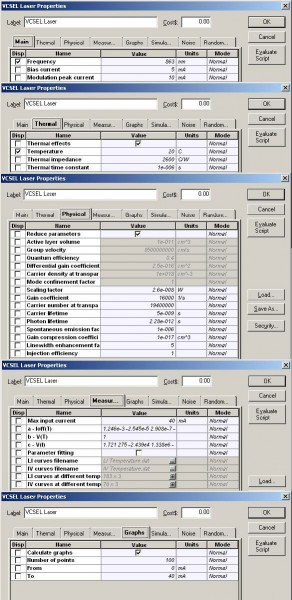Figure 2: VCSEL parameters for the 863 nm laser

## Graphs

The user should also provide the LI and IV curves for the device.

For this example, the data from Figures 1 and 2 from  are used.

By default, the component already has the data stored internally and this will not be necessary for the 863 nm laser:

• Go to OptiSystem project browser.
• Select the “VCSEL Laser” component.
• Select the “Graphs” folder.
• Double-click on the “Measured LI curve” (Figure 3).

The graph should be the same as the graph in Figure 2 from .

Repeat the same steps for the second graph “Measured IV curve”. The graph should be the same as Figure 3 from .Figure 3: Graphs folder for the VCSEL laser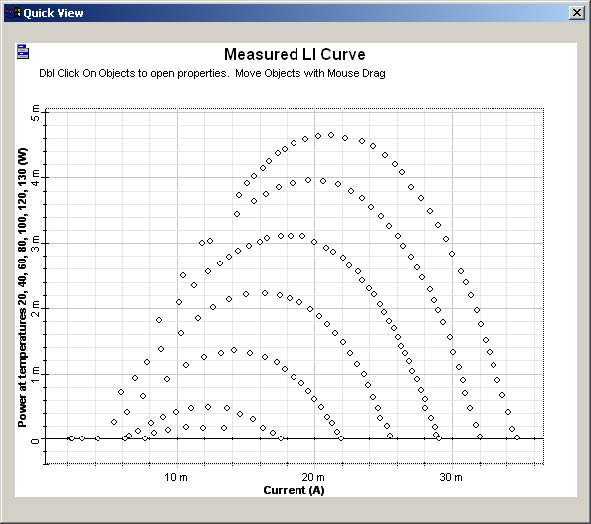Figure 4: LI curve graphs for the VCSEL laser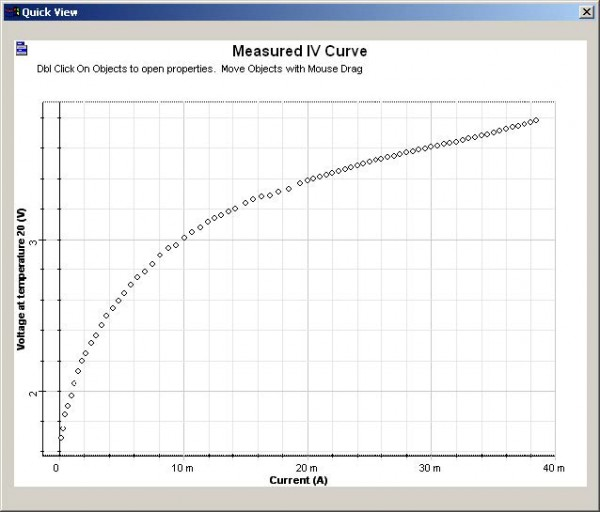Figure 5: IV curve graphs for the VCSEL laser

## Parameter sweep

The next step is to simulate for different temperatures in order to compare the measured curves from Figure 4 and Figure 5 with the calculation results.

Create a sweep with the laser parameter Temperature for the values of 20, 40, 60, 80,100, 120 and 130° Celsius (Figure 6).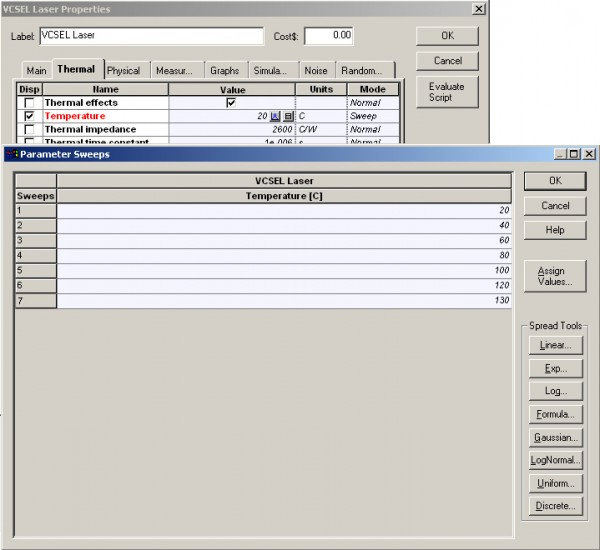Figure 6: Temperature parameter sweep

## Running the simulation

We can run this simulation and analyze the results:

• To run the simulation, you can go to the File menu and select Calculate. You can also press Control+F5 or use the calculate button in the toolbar. After you select Calculate, the calculation dialog box should appear.
• In the calculation dialog box, press the Play button. The calculation should take a few seconds.

## Viewing the results

Because we are interested in the LI and IV curves from the simulation, we can select the graphs from the project browser: “LI curve” and “IV curve”.

To compare the results with the measurements, repeat the same steps to see the measured graphs. However, instead of double-clicking on the graphs, press the Ctrl key and the left mouse button to select the “LI curve” and the “LI curve measured”:Figure 7: Multiple graphs selection

Now click the right button of the mouse and select Quick View.

The graph for the LI curve is presented in the Figure 8.

As you can see, the simulation is very close to the original measurements, as expected by .

Repeat the same steps for the IV curve, and the graph should look like Figure 9.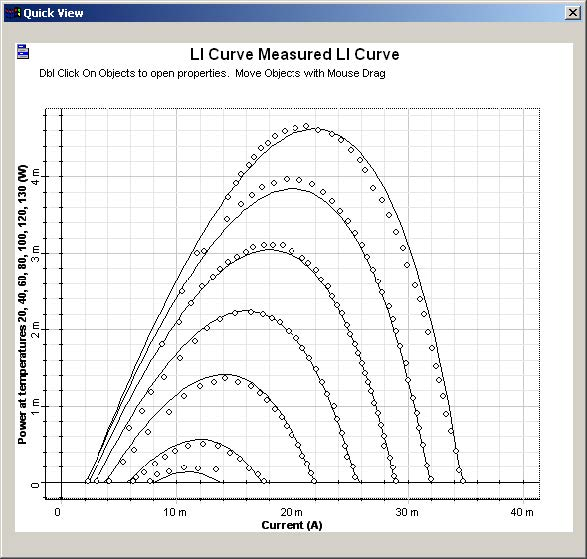Figure 8: LI curves: The dotted line is the measurement.Figure 9: IV curves: The dotted line is the measurement.

## Parameter fitting

The next step is to enable Parameter fitting and run the simulation again.

This time, the component will use the previous parameters as a first guess for the parameter-fitting engine and calculate new parameters:

• In the VCSEL laser parameters dialog box, Measurements tab, enable the parameter Parameter fitting.
• Close the dialog box and run the simulation

After the simulation is finished, you can compare the LI and IV graphs with Figure 8 and Figure 9. The graphs will be very similar, however the laser parameters are different.

After the simulation is finished, you can compare the LI and IV graphs with Figure 8 and Figure 9. The graphs will be very similar, however the laser parameters are different.

## Second device: 683 nm laser

Parameter setup

The laser parameters of OptiSystem VCSEL component should be the same as the 683 nm VCSEL from .

 Parameter Value Frequency 683 nm Thermal impedance 9800 Thermal time constant 1e-006 Scaling factor 2.6e-008 Gain coefficient 16000 Carrier number at transparency 16540000 Carrier lifetime 5e-009 Photon lifetime 2.064e-012 Spontaneous emission factor 1e-006 Injection efficiency 1 Max input current 5 a – Ioff(T) -2.734e-4, -2.125e-5, 1.837e-7, 3.183e-10 b – V(T) 0.829, -1.007e-3, 6.594e-6, -2.18e-8 c – V(I) 1.721 275, -2.439e4, 1.338e6, -4.154e7, 6.683e8, -4.296e9

Table 2: 683 nm VCSEL parameters

To load the measurements from this device, we should enable the Parameter fitting parameter and import the measured LI and IV curves.

• In the Measurements tab, click on the parameter LI curves filename.
• Load the file “Measured LI 683 VCSEL.dat”.
• Click on the parameter IV curves filename.
• Load the file “Measured IV 683 VCSEL.dat”.
• Disable the parameter Parameter fitting.

Figure 10 presents the main VCSEL parameters for the 683 nm laser, after loading the files and disabling the parameter fitting: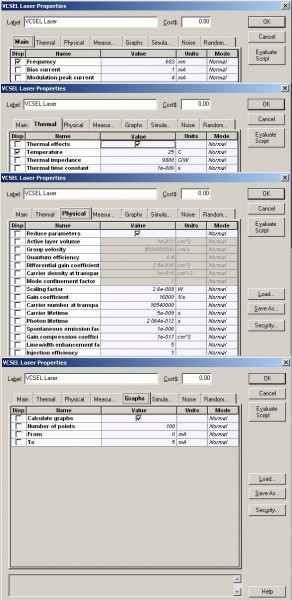Figure 10: VCSEL parameters for the 683 nm laser

## Graphs

The graphs for “Measured LI curve” should be the same as Figure 4 from .

See Figure 11.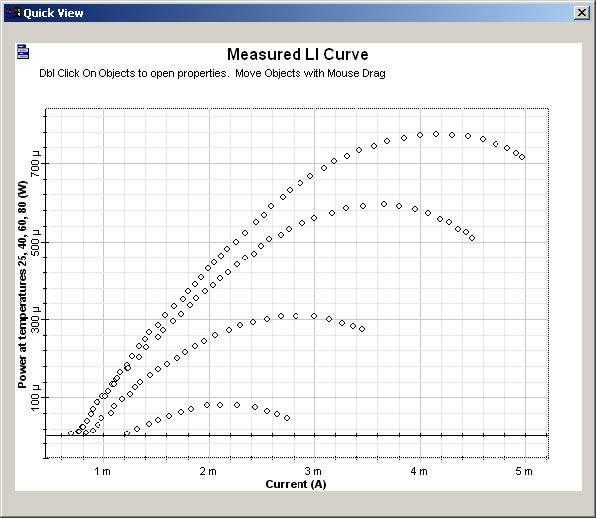Figure 11: LI curve graphs for the VCSEL laser

## Parameter sweep

The next step is to simulate for different temperatures in order to compare the measured curves from Figure 11 with the calculation results.

Create a sweep with the laser parameter Temperature for the values of 25, 40, 60 and 80° Celsius. Now run the simulation.

## Viewing the results

The graph for the LI curve is presented in the Figure 12.

As you can see, the simulation is very close to the original measurements for 25, 40 and 60° Celsius, as expected by .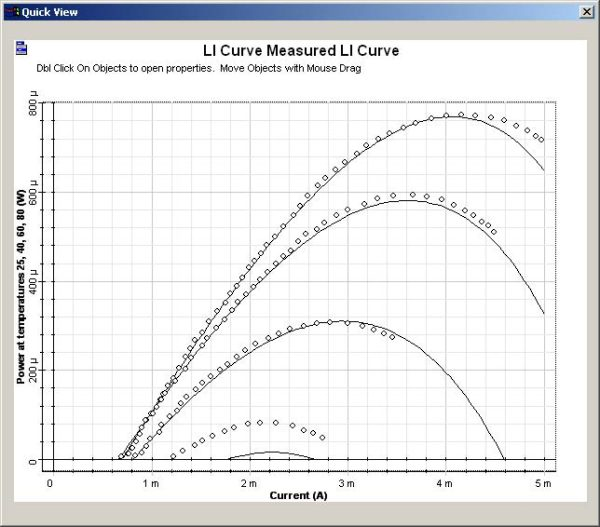Figure 12: LI curves: The dotted line is the measurement.

## Parameter fitting

The next step is to enable Parameter fitting and run the simulation again.

This time the component will use the previous parameters as a first guess for the parameter-fitting engine and calculate new parameters.

The resultant graph is similar to theFigure 12, and the new parameters are available as results in the project browser.

## Third device: 1550 nm laser

Parameter setup

The system parameters for this project should be the same as .

To compare the results, we will generate one single pulse at 2.4 GBs.

This means that we should change the global parameters. Also, we should use the same rise and fall times for the NRZ pulse generator and replace the PRBS generator with a user-defined bit sequence generator:

• In the global parameters dialog box, change the parameter Bit rate to 2.4e9 and the parameter Sequence length to 16 (Figure 13).
• Replace the PRBS bit sequence generator with the “User Defined Bit Sequence Generator” from “Default/Transmitters Library/Bit Sequence Generators”. Connect the components according to Figure 14.
• In the User Defined Bit Sequence Generator parameter dialog box, change the parameter Bit sequence to “00000010000000” (Figure 15).
• In the NRZ Pulse Generator parameter dialog box, change the parameters Rise time and Fall time to 0.25 (Figure 16).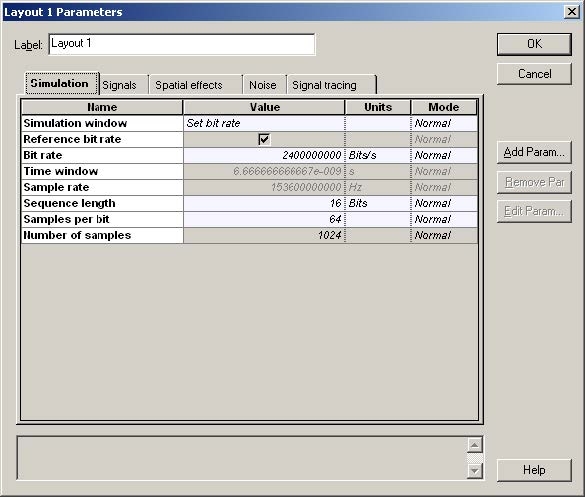Figure 13: Global parameters for laser 1550 nm setupFigure 14: 1550 nm transmitter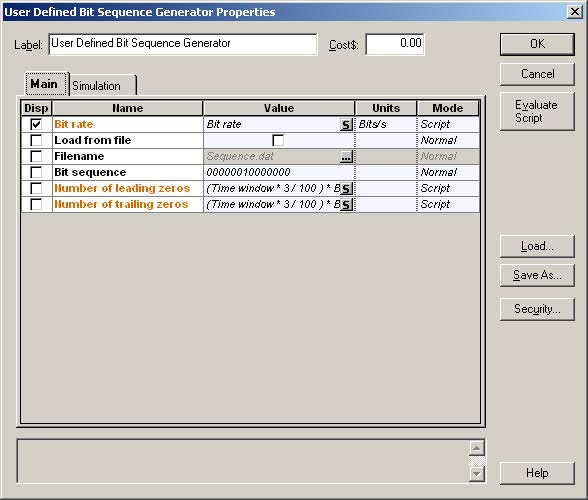Figure 15: Bit sequence generator parameters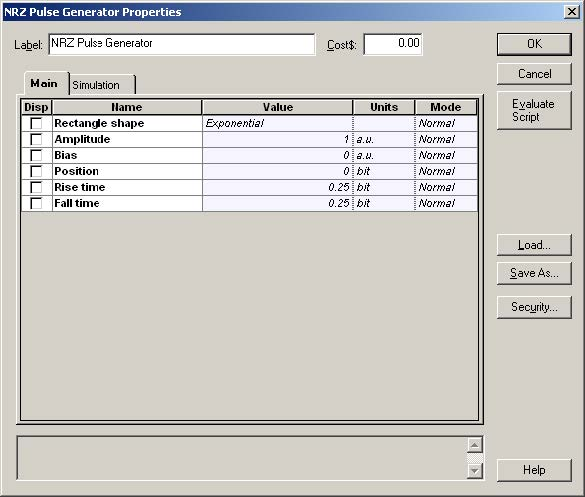Figure 16: NRZ pulse generator parameters

The laser parameters of component should be the same as the laser from .

 Parameter Value Frequency 1550 nm Bias current 38 Modulation peak current 28 Thermal effects False Reduce parameters False Active layer volume 1.5e-010 Group velocity 8500000000 Quantum efficiency 0.4 Differential gain coefficient 2.5e-016 Carrier density at transparency 1e+018 Mode confinement factor 0.4 Carrier lifetime 1e-009 Photon lifetime 3e-012 Spontaneous emission factor 3e-005 Gain compression coefficient 1e-017 Linewidth enhancement factor 5 Injection efficiency 1

Table 3: 1550 nm laser parameters

For this particular device, we will not use the thermal effects and we will provide the same parameters as in .

This means that the parameters Thermal effects and Reduce parameters will be disabled. Because the thermal effects are disabled, the parameters from the Measurements tab will not be used in the simulation.

Figure 17 presents the main VCSEL parameters for the 1550 nm laser: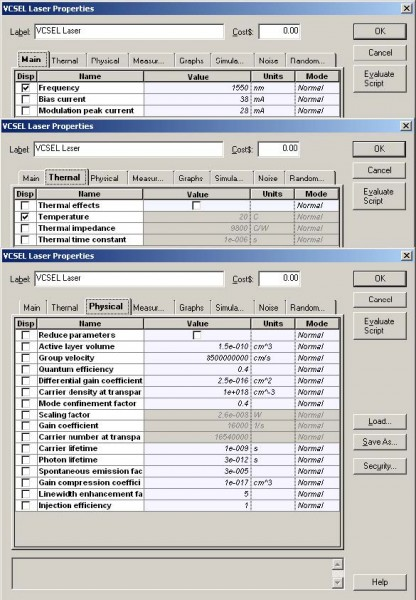Figure 17: Parameters for the 1550 nm laser

## Parameter sweep

The next step is to simulate for different values of gain compression coefficients in order to compare the results from  with the calculation results.

Create a sweep with the laser parameter Gain compression coefficient for the values of 1×10-17 and 5×10-17.

Run the simulation.

## Viewing the results

First we should compare the shape of the electrical pulse with Figure 2a from .

Double click on the “Oscilloscope Visualizer” in the layout.

Figure 18 presents the applied pulse at the laser input. The pulse is very similar to the one shown in Figure 2a from . The amplitudes of the pulses are slightly different because OptiSystem generated a normalized pulse.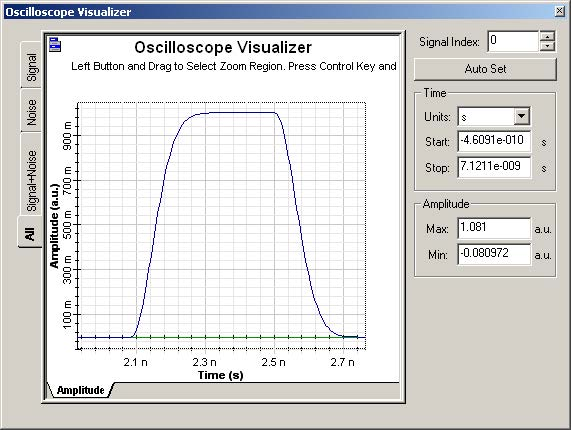Figure 18: Applied pulse at the laser input

The next step is to compare the optical pulse at the laser output with Figure 2b from .

Using the project browser, select the ‘Signal power” graph from the “Optical Time Domain Visualizer” (Figure 19).

Figure 20 presents the optical pulses at the laser output. The results are very similar to the Figure 2b from .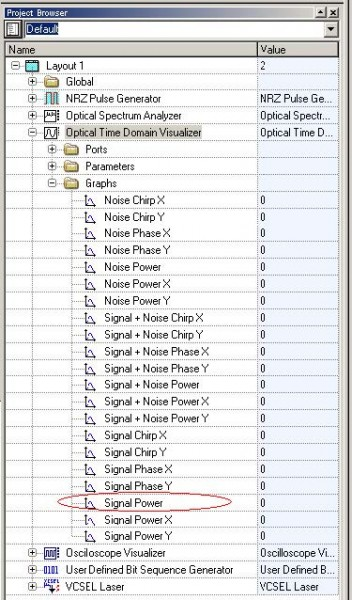Figure 19: Selecting the signal power graphs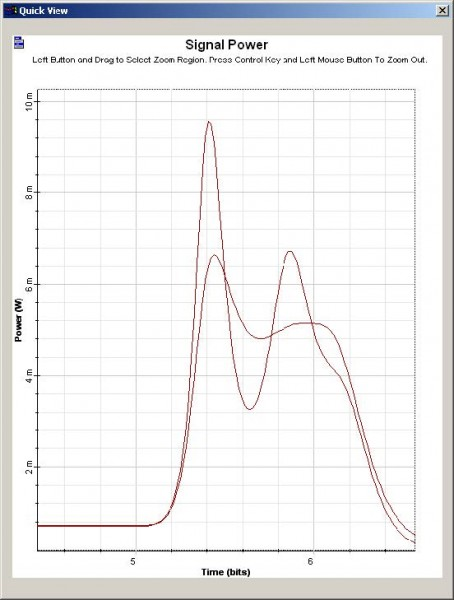Figure 20: Optical pulse at the laser output for different values of gain compression coefficients

References

J. C. Cartledge and G. S. Burley, “The Effect of the Laser Chirping on Lightwave System Performance”, J. Lightwave Technology, vol. 7, pp. 568-573, March 1989.

P. V. Mena, J. J. Morikuni, S. M. Kang, A. V. Harton and K. W. Wyatt, “A Simple Rate-Equation-Based Thermal VCSEL Model”, J. Lightwave Technology, vol. 17, pp. 865-872, May 1999.